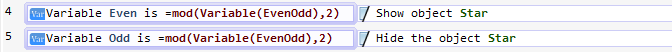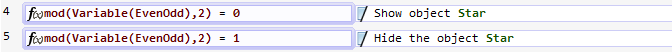# Even number or odd number condition for a variable?

how to check if a value of a variable is even or odd?

You can use this expression :

``mod(Variable(myVar), 2)``

(it’s the modulo by 2).
If it’s equal to 1, the number is odd, otherwise, if it’s equal to 0, the number is even.

I have tried a little scene in which I have add an action. Do = mod(Variable(Var),2) to the variable Var. But it is not working. how this thing really works

Do that to another variable because you’re actually detroying the content of your variable…

It will be easier if you explain it on this file. Please!
EvenOdd.zip (3.18 KB)

if you help me with this file it will be easier for me to built my game. Please Help me!

The variable EvenOdd will be 0, 1, 2, 3, …, etc. But the expression “mod(Variable(Even), 2)” and “mod(Variable(Odd), 2)” will be 0 and 1 respectively, so after two seconds your conditions “variable EvenOdd is = mod(Variable(Even), 2)” and “variable EvenOdd is = mod(Variable(Odd), 2)” will never be trueYou have to use the function “mod” on the EvenOdd variable:
EvenOdd = 0 ==> mod(EvenOdd, 2) = 0
EvenOdd = 1 ==> mod(EvenOdd, 2) = 1
EvenOdd = 2 ==> mod(EvenOdd, 2) = 0
EvenOdd = 3 ==> mod(EvenOdd, 2) = 1
In other words, you can do it:Or:how to do f(x)mod(Variable(EvenOdd).2) as an action?

This condition just test the expressions, if you want to use the expression “mod(Variable(EvenOdd))” in an action… use it!I mean, in any field that needs a numerical expression, you can put this “mod” function